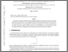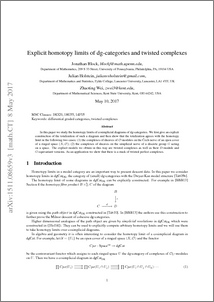# Explicit homotopy limits of dg-categories and twisted complexes

Block, Jonathan and Holstein, Julian V. S. and Wei, Zhaoting (2017) Explicit homotopy limits of dg-categories and twisted complexes. Homology, Homotopy and Applications, 19 (2). pp. 343-371. ISSN 1532-0073Preview
PDF (1511 (2).08659v3)
1511_2_.08659v3.pdf - Accepted Version
In this paper we study the homotopy limits of cosimplicial diagrams of dg-categories. We first give an explicit construction of the totalization of such a diagram and then show that the totalization agrees with the homotopy limit in the following two cases: (1) the complexes of sheaves of $\mathcal O$-modules on the \v{C}ech nerve of an open cover of a ringed space $(X, \mathcal O)$; (2) the complexes of sheaves on the simplicial nerve of a discrete group $G$ acting on a space. The explicit models we obtain in this way are twisted complexes as well as their $D$-module and $G$-equivariant versions. As an application we show that there is a stack of twisted perfect complexes.View Item